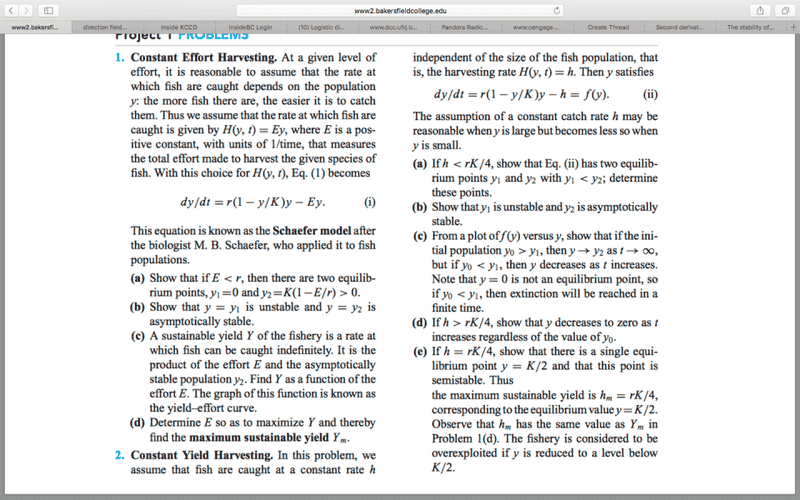# Differential equation: Autonomous equation question

## Homework StatementI'm stuck on Question #2 part A/B

## Homework Equations

y'=r(1-y/k)y-h=y^2-ky+kh/r
y''=2y-k
Roots for y'= (k+/-sqrt(k^2-4kh/r))/2 Im assuming the positive root is y2
h<rk/4
[/B]

## The Attempt at a Solution

on part a I'm getting the roots to be y2=(K+sqrt(k^2-4kh/r))/2 and y1=(K-sqrt(k^2-4kh/r))/2... I then got the second derivative of the function to be y''=2y-k and when i plug in the equilibrium equations to check which one is stable and unstable I get for y2 that it is unstable and for y1 it's stable but I know that it should be the reverse... unless somehow y2 is less than y1. Or I'm mislead in thinking that y''<0 is stable and y''>0 is unstable (in question #1 that is how it seemed to work out)

## Answers and Replies

epenguin
Homework Helper
Gold Member
Forget y'' - you only need to think about y'